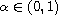Electron. J. Differential Equations, Vol. 2017 (2017), No. 44, pp. 1-12.

### Moving-boundary problems for the time-fractional diffusion equation Sabrina D. Roscani

Abstract:
We consider a one-dimensional moving-boundary problem for the time-fractional diffusion equation. The time-fractional derivative of orderis taken in the sense of Caputo. We study the asymptotic behaivor, as t tends to infinity, of a general solution by using a fractional weak maximum principle. Also, we give some particular exact solutions in terms of Wright functions.

Submitted August 26, 2016. Published February 14, 2017.
Math Subject Classifications: 26A33, 35R37, 33E12, 33E20.
Key Words: Fractional diffusion equation; Caputo derivative; moving-boundary problem; maximum principle; asymptotic behaivor.

Show me the PDF file (248 KB), TEX file for this article.Sabrina D. Roscani CONICET - Departamento de Matemática FCE Universidad Austral, Paraguay 1950 S2000FZF Rosario, Argentina email: sabrinaroscani@gmail.com

Return to the EJDE web page